# Motion problem

From Levíc to Košíc go car at speed 81 km/h.
From Košíc to Levíc go another car at speed 69 km/h.

How many minutes before the meeting will be cars 27 km away?

Result

t =  10.8 min

#### Solution:Leave us a comment of example and its solution (i.e. if it is still somewhat unclear...):Be the first to comment!#### To solve this example are needed these knowledge from mathematics:

Need help calculate sum, simplify or multiply fractions? Try our fraction calculator. Do you have a linear equation or system of equations and looking for its solution? Or do you have quadratic equation? Do you want to convert length units?

## Next similar examples:

1. Ping time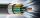Calculate theoretical ping time between Orlando and Shenzhen which is 14102 km distant. Ping time measures the round-trip time for small messages sent from the origin to a destination that is echoed back to the source. The name comes from active sonar term
2. Earth and Sun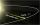Earth is 150 million km away from the Sun, 300,000 km of sunlight travels in 1 second. How long will the light come from the Sun to the Earth?
3. Bob traveled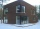Bob traveled 20 meters to Dave's house. He arrived in 3 minutes. What was his average speed for the trip.
4. Wood dividingWhich equation calculates the number of 1/3-foot pieces that can be cut from a piece of wood that is 7 feet long?
5. PipeSteel pipe has a length 2.5 meters. About how many decimetres is 1/3 less than 4/8 of this steel pipe?
6. CakesGrandmother baked cakes. Half of its was poppy, quarter with plum jam and 16 cheesecakes. How many cakes she baked in total?
7. PlayingHow long have we trained on the pitch when we know that the warm-up took 10 minutes, we trained passes for one-third of the time and we played football half the time?
8. Equation 20In given equation: 8/9-4/5=2/9+x, find x
9. RatioIncrease in the ratio 20:4 number 18.5.
10. TeacherTeacher Rem bought 360 pieces of cupcakes for the outreach program of their school. 5/9 of the cupcakes were chocolate flavor and 1/4 wete pandan flavor and the rest were vanilla flavor. How much more chocolate flavor cupcakes than vanilla flavor?
11. Simple equationSolve for x: 3(x + 2) = x - 18
12. SeamstressThe seamstress cut the fabric into 3 parts. The first part was the eighth fabric, the second part was three-fifths of the fabric and the third part had a length of 66 cm. Calculate the original length of the fabric.
13. Write 2Write 791 thousandths as fraction in expanded form.
14. Geometric plan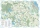At what scale the building plan if one side of the building is 45m long and 12mm long on a plan?
15. Bar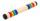Half of the bar is green, the third is yellow and the remaining 40cm is red. How long is a bar?
16. Rope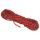One half of rope use Mam for packaging, half of the rest got son, half of the rest he took father and two fifths of what remained, got daughter. Remained 143 cm of rope. How long was rope at the beginning?
17. EquationFind x: x + 1/2 = 1/3